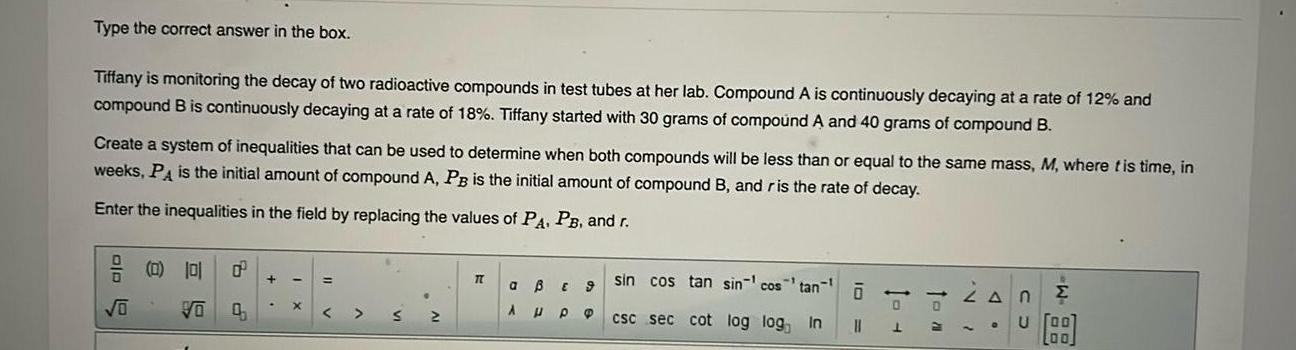Question:

# Type the correct answer in the box Tiffany is monitoring the

Last updated: 11/19/2023Type the correct answer in the box Tiffany is monitoring the decay of two radioactive compounds in test tubes at her lab Compound A is continuously decaying at a rate of 12 and compound B is continuously decaying at a rate of 18 Tiffany started with 30 grams of compound A and 40 grams of compound B Create a system of inequalities that can be used to determine when both compounds will be less than or equal to the same mass M where t is time in weeks PA is the initial amount of compound A PB is the initial amount of compound B and ris the rate of decay Enter the inequalities in the field by replacing the values of PA PB and r olo O 0 101 0 Vo 0 X TT a B A P sin cos tan sin cos tan 9 PO csc sec cot log log In 0 11 0 L VO A U 00 L00